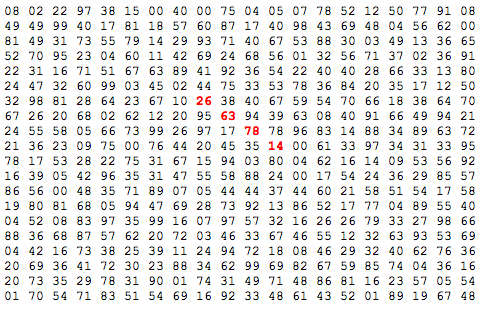# Functional Programming: Shaping the data to fit a function

As I mentioned in my last post I’ve been working on Project Euler problem 11 and one thing I noticed was that I was shaping the data around a windowed function since it seemed to fit the problem quite well.

Problem 11 is defined like so:

In the 20x20 grid below, four numbers along a diagonal line have been marked in red.The product of these numbers is 26 63 78 14 = 1788696. What is the greatest product of four adjacent numbers in any direction (up, down, left, right, or diagonally) in the 20x20 grid?

I needed to get all the horizontals, verticals and diagonals into collections that I could then apply the function to and get the four adjacent numbers required by the problem.

That was reasonably easy for the horizontals and verticals:

``````
leftToRight = concatMap (windowed 4) grid
topToBottom = concatMap (windowed 4) [[row !! (n-1) | row <- grid] | n <- [1..length grid]]
``````
``````
-- shortened for brevity
grid :: [[Int]]
grid = [[08,02,22,97,38,15,00,40,00,75,04,05,07,78,52,12,50,77,91,08],
....
[01,70,54,71,83,51,54,69,16,92,33,48,61,43,52,01,89,19,67,48]]
``````

In the first case we’re just running a map operation over each row and converting it into groups of 4 adjacent elements. By using concatMap we can ensure that the result is flattened back into a single collection.

To get the top to bottom values I had to first create a new array of arrays built up using values at the same column index in each row before applying the windowed function.

I found it much more difficult to get the diagonals into the right shape but luckily I bumped into Uday who helped me figure it out.

We started off by working out how to calculate all the numbers diagonally left to right from a specific point which resulted in this function:

``````
diagonalAt :: Int -> Int -> [[Int]] -> [Int]
diagonalAt x y grid = [findValue (x+z) (y+z) grid | z <- [0..(length grid)], hasItem grid (x + z) (y + z)]
``````

We had to add a hasItem function so that we didn’t try and call findValue on a position which didn’t exist on the grid:

``````
hasItemSingle :: [a] -> Int -> Bool
hasItemSingle array position = position >= 0 && (length array - 1) >= position

hasItem :: [[a]] -> Int -> Int -> Bool
hasItem array x y = hasItemSingle array x && hasItemSingle (array !! x) y
``````

findValue is just used to find the value at an (x,y) location:

``````
findValue :: Int -> Int -> [[Int]] -> Int
findValue x y grid = (grid !! x) !! y
``````

Now we had the ability to find out one diagonal we needed to find all the diagonals on the grid which we did like this:

``````
diagonals :: [[Int]] -> [[Int]]
diagonals grid = filter (\x -> length x > 4) . map (\(x,y) -> diagonalAt x y grid) \$ (diagonalPositions grid)

diagonalPositions :: [[Int]] -> [(Int, Int)]
diagonalPositions grid = [ (x,y) | x <- [0..(length grid)], y <- [0..length \$ grid !! 0 ]]

diagonalValues = concatMap (windowed 4) (diagonals grid)
``````

diagonalPositions creates a sequence of pairs which contain all the different positions in the grid where we want to try and find a diagonal from. We then map over that in diagonals and find the diagonal from each of those places.

We then had to do the same to find diagonals running from right to left:

``````
diagonalUpwaysAt :: Int -> Int -> [[Int]] -> [Int]
diagonalUpwaysAt x y grid = [findValue (x+z) (y+(z * (-1))) grid | z <- [0,-1..(length grid * (-1))], hasItem grid (x + z) (y + (z *(-1)))

diagonalsUp :: [[Int]] -> [[Int]]
diagonalsUp grid = filter (\x -> length x > 4) . map (\(x,y) -> diagonalUpwaysAt x y grid) \$ (diagonalPositions grid)

diagonalUpValues = concatMap (windowed 4) (diagonalsUp grid)
``````

In this case instead of incrementing our position by 1 on the x and y axes we needed to decrement our position on the y axis so it’d find the value one row above us.

To find the maximum product of 4 adjacent numbers in the grid we need to do the following:

``````
problem_11 = maximum \$ map product \$ topToBottom ++ leftToRight ++ diagonalValues ++ diagonalUpValues
``````
``````
> problem_11
70600674
``````

Obviously there’s a lot of duplication in this solution so another exercise for me is to try and make it a bit more concise!

I think I picked up this way of solving problems from playing around with clojure last year and it seems quite neat although I’m sure it might not seem that intuitive to someone who hadn’t seen it before.

The code for the whole solution is available as a gist.